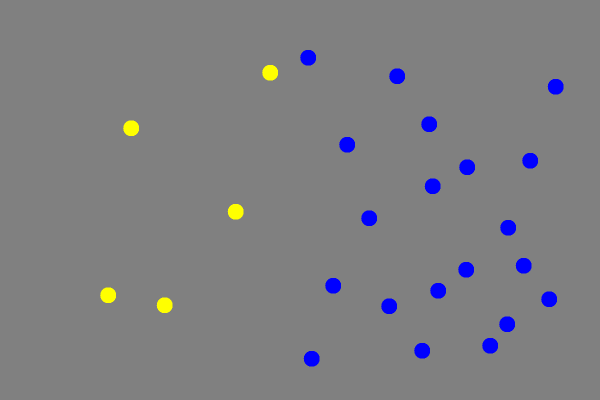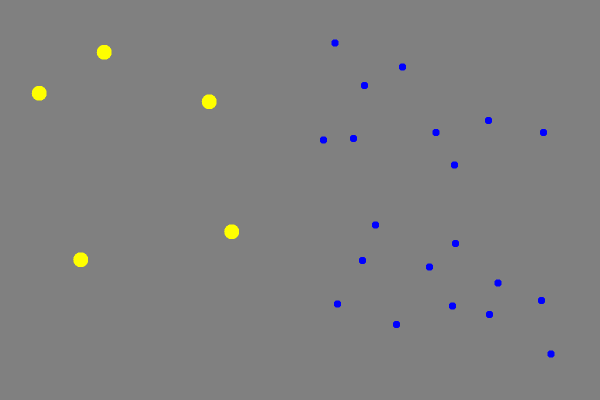# Subject ID

-talk about the point of subject id here

# Age

-talk about age. importance. does it matter if you put the wrong age. consequences of doing so. etc.

# How Ratio Bins Are Calculated From Age And Difficulty

If the user has not entered customized settings under Advanced Options on the main panel then the program will generate appropriate settings for numerosity ratio and display time. It does this by taking the users age and selected difficulty, adjusting the age based on the difficulty, then finding appropriate values for numerosity ratio and display time using this adjusted age. The following sections show exactly how adjusted age, numerosity ratio, and display time are calculated.

• Very Easy Difficulty
• If user's age is between 0 and 20, then the formula .5 * age is used. If the user is between 20 and 40, then 3/20 * age + 7 is used. Otherwise, an adjusted age of 13 is used. In all subsequent algorithms, this adjusted age is used instead of the real age. This casuses the numerosity ratio and display time to scale with age and difficulty.
• Easy Difficulty
• If user's age is between 0 and 20, then the formula 3/5 * age is used. If the user is between 20 and 40, then 3/20 * age + 9 is used. Otherwise, an adjusted age of 15 is used.
• Medium Difficulty
• On medium difficulty, the user's real age is used in all calculations. This means that a 20 year old will have an adjusted age of 20, a 40 year old will have an adjusted age of 40, etc.
• Hard Difficulty
• On hard difficulty, the adjusted age is given by the formula 4.116713430316e-01 + 1.111762903248e+00 * actualAge + 9.684975424473e-03 * actualAge^2. Note that at this difficulty, this formula is always used, unlike for very easy and easy difficulty, in which after 40 the same value is used.
• Very Hard Difficulty
• On very had difficulty, the adjusted age is given by the formula 4.755221349509e-01 + 1.477883254935e+00 * actualAge+ 1.382024390740e-02 * actualAge^2. This formula is used for all input ages.

## Numerosity Ratio

Once the adjusted age has been calculated by the above formulas, it is run through a function to determine appropriate numerosity ratios based on the adjusted age and the difficulty. When calculating the numerosity ratios, the weber fraction is used. The [Fraction] is the amount of change in a stimulus relative to the background "noise" in the stimulus. The important thing about the weber fraction is that it is roughly constant for a certain stimulus. In vision lab experiments, the weber fraction for panamath experiments is approximately weberFraction = adjustedAge ^ -.55. This value is used to calculate scaled values for numerosity ratio according to the following rules:

• If the adjusted age is less than 40 or is on very hard or hard difficulty, then the following four values are used as the numerosity ratios.
• (.75*w) + 1
• (1.25*w) + 1
• (2*w) + 1
• 2*(w + 1)
• If the adjusted age is greater than 40 and the user is on very easy, easy, or medium difficulty, then the following values are used for numerosity ratio, based on difficulty.
• Very Easy Difficulty
• 1.5
• 1.8
• 2.8
• 4.0
• Easy Difficulty
• 1.4
• 1.5
• 2.5
• 3.5
• Medium Difficulty
• 1.2
• 1.3
• 1.8
• 3.0

## Display Time

Display time is the simplest to calculate. It is based only on adjusted age, and is calculated according to the following rules:

• If the user's adjusted age is between 0 and 20, it is calculated according to the formula 3.23713 * e^(-0.0892973 * adjustedAge) + 0.0573327.
• If adjusted age is between 20 and 40, a display time of .6 seconds is used
• If adjusted age is between 40 and 70, display time is calculated according to the formula 0.0467 * adjustedAge - 1.2667
• If adjusted age is greater than 70, then display time is set to 2 seconds

## How Size Controlling Works

Size control changes the average size of the dots in relation to the number of dots of each color.

Without size control, it would be possible to get most of the trials correct by simply noting which color fills up more area on the screen. A size control of 0, Non Size-Controlled, creates dots with the same average size for both sets of dots, while a negative size control creates smaller dots for the set with more dots, and a positive size control creates larger dots for the set with more dots.

A size control of -1,Size-Controlled, changes the average size of each dot set so that the area of the screen filled by each dot set is approximately equal.

If all the trials in a test used the same size control, it would be possible to get most of the trials correct by paying attention to either the average sizes of the dots or the area of the screen filled with each color. By using a combination of different size controls, we can ensure that the area and size of the dots will not reveal the correct answer. The size control number, which can be adjusted from -3 to 1 in the customize panel of the advanced settings panel, is the exponent to which the ratio of the number of dots is raised to obtain the ratio of the average sizes of dots.

### Non Size-Controlled

In a non size-controlled experiment, size control is 0. The ratio of the average sizes of the dots is calculated to be (numerosity ratio)0, which means that the ratio of the average sizes of the two dot sets is 1 for any numerosity ratio. This results in total area of each color being proportional to the number of dots.### Size-Controlled

In a size-controlled experiment, size control is -1. The ratio of the average sizes of the dots is (numerosity ratio)-1. With this setting, the total area that a one dot set fills will be the same as the other dot set, pixel ratio of the two colors is approximately 1:1.
In the example below, there are 5 yellow dots and 20 blue dots. The ratio of the sizes of dots is (1/4)-1 or (4/1). The yellow dots to blue dots average size ratio is 4:1.### Anti-Correlated

In an anti-correlated experiment, size control is -2. The ratio of the average sizes of the dots is (numerosity ratio)-2. With this setting, the total area that the dot set with less dots fills will be more than the area of the dot set with more dots.\\ In the example below, there are 5 yellow dots and 20 blue dots. The ratio of the sizes of dots is (1/4)-2 or (16/1). The yellow dots to blue dots average size ratio is 16:1.\\ Sizecontrol-2.png?600x400

# Basics of the Program

-talk about basics of the actual program, including how to go between trials, how to respond, etc. very basic stuff.

## Questions

If you have any questions on how to use Panamath, please send us a message or e-mail us directly at panamath@panamath.org.